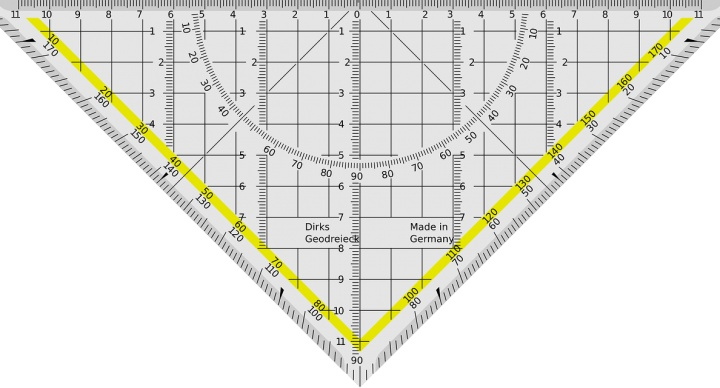# Pre-Algebra – SE### Course Overview

Acellus Pre-Algebra-SE was developed to provide special education students with an introduction to algebraic concepts, including the basic principles, rules, and operations of working with expressions containing variables. It also provides a strong foundation in basic mathematical concepts to prepare them for Acellus Algebra-SE. Course topics include:
• Whole Number Operations
• Order of Operations
• Fractions/Decimals/Percentages
• Basic Probabilities and Statistics
• Basic Geometry
• Integer Operations
• Basic Graphing
• Basic One- and Two-Step Equations
Acellus Pre-Algebra-SE is based on NCTM standards. It is taught by award-winning Acellus Master Teacher, Patrick Mara.This course was developed by the International Academy of Science. Learn More

### Scope and Sequence

Unit 1 – Introduction and Basic Foundation This unit covers addition, subtraction, multiplication, and division of whole numbers, prime and composite numbers, greatest common factors, prime factorization, place value, estimating, order of operations, powers of ten, and formulas and variables. Unit 2 – Fractions This unit discusses ratios, rates, proportions, improper and mixed fractions, simplifying fractions, least common multiples, adding, subtracting, multiplying, and dividing fractions and mixed numbers. Unit 3 – Decimals, Fractions, and Percents This unit covers adding, subtracting, and multiplying decimals, as well as dividing decimals by whole numbers, comparing and ordering decimals, forms and types of percent, and simple interest. Unit 4 – Probability and Statistics This unit covers basic probability, mean, median, mode, range, frequency tables, bar graphs and broken-line graphs. Unit 5 – Geometry Basics This unit discusses geometry symbols, planes, points, lines, and angles. It also covers triangles, quadrilaterals, and other polygons, with particular emphasis on triangles. This unit is followed by the Mid-Term Review and Exam Unit 6 – More Geometry This unit discusses length and perimeter, area in two-dimensional shapes, and volume in three-dimensional figures. Also covered are square roots, the Pythagorean Theorem, and circles. Unit 7 – Pre-Algebra This unit covers comparing and ordering, adding, subtracting, multiplying and dividing integers, absolute value, and order of operations. Also covered are the properties of mathematics, and basic graphing including ordered pairs and the coordinate plane. Unit 8 – Beginning Algebra This unit covers comparing, ordering, adding, subtracting, multiplying, and dividing rational numbers, as well as expressions and equations, order of operations with and without variables, and the meaning of algebraic symbols. Also discussed are one- and two-step equations, decimals and fractions, formulas, and literal equations. This unit is followed by the Final Review and Exam